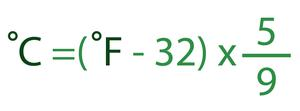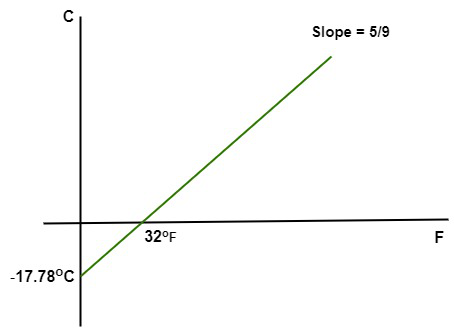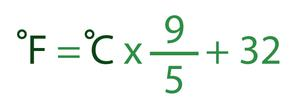# Fahrenheit to Celsius Formula

• Last Updated : 18 Aug, 2022

Fahrenheit to Celsius formula is the conversion of the units to measure temperature. It is seen in daily lives that when the temperature of anything, say water, is measured, the unit is denoted as Celsius. The body temperature is often denoted in Fahrenheit. However, both temperature scales are easily convertible to each other. Before learning how to convert Fahrenheit to celsius, let’s learn more about the terms Fahrenheit and celsius units in detail.

## Fahrenheit

Fahrenheit is a scale of temperature. Its unit is the degree Fahrenheit (°F). This unit is named after physicist Daniel Gabriel Fahrenheit who first proposed this temperature scale in 1724. On the Fahrenheit scale, the water freezes at 32°F and boils at 212°F under normal conditions. Fahrenheit is also the U.S. customary measurement unit of temperature.

## Celsius

Celsius is also a scale of temperature. Its unit is the degree Celsius (°C). This unit was named after astronomer Anders Celsius in 1948. On the Celsius scale, the water freezes at 0°C and boils at 100°C under normal conditions. Almost the whole world uses Celsius for temperature measurement aside from the U.S. Celsius is sometimes referred to as Centigrade.

## What is Fahrenheit to Celsius Formula?

To convert Fahrenheit to celsius, the formula used is °C = 5/9(°F – 32). Using Fahrenheit to Celsius formula (F to C formula), the temperature in Fahrenheit can easily be converted into Celsius.Example: Convert 80 Fahrenheit to Celsius.

Solution:

Given, F = 80°

Using the formula, °C = 5/9(°F – 32)

C = 5/9(80 – 32)

C = 26.67°C

Hence, 80°F = 26.67°C

As mentioned above, the freezing point and boiling point in the Fahrenheit scale are 32°F and 212°F, respectively. Similarly, for Celsius, it is 0°C and 100°C, respectively. Let’s assume a graph in which the X-axis corresponds to Fahrenheit and the Y-axis corresponds to Celsius.Now calculate the slope of this graph using the formula: Slope (m) = Δy/Δx.

Consider y1 = 0, y2 = -17.78, x1 = 32, x2 = 0

Slope (m) = Δy/Δx

= 17.78/32

= 5/9

After this, we will use point-slope formula y – y1 = m(x – x1). Instead of using y and x as variables, we will be using C and F respectively. So, the formula becomes C – C1 = m(F – F1).

Now, let’s put in the values,

°C – 0 = 5/9(°F – 32)

°C = 5/9(°F – 32)

## Fahrenheit to Celsius Conversion

Below given steps can be helpful in Fahrenheit to Celsius conversion:

1. First of all, whatever value is given in Fahrenheit, subtract 32 from the value.
2. Now the value should be multiplied by 5.
3. The last step is to divide the value obtained in step 2 by 9.

## Celsius to Fahrenheit Formula

In order to convert Celsius to Fahrenheit, a specific formula is used. Celsius to Fahrenheit formula or C to F is obtained from the above given Fahrenheit to celsius formula, and hence, Celsius to Fahrenheit formula will be °F = 9/5°C + 32. By using the formula, we can convert any temperature given in Celsius to Fahrenheit.Example: Convert 35.5 Celsius to Fahrenheit?

Solution:

The temperature given in celsius is 35.5 C; we will be using the formula,

°F = 9/5°C + 32

°F = 9/5 (35.5) + 32

°F = 63.9 + 32

°F = 95.9

### Fahrenheit to Celsius Conversion Table

A table for temperature can be formed for any temperature unit using the temperature conversion formula. Here, the above formula is used to make the table. Temperature values converted from Fahrenheit to celsius are shown below,

## Solved Examples on Fahrenheit to Celsius Formula

Question 1: Convert 80 Fahrenheit to Celsius.

Solution:

Given, F = 80°

Using F to C formula, °C = 5/9(°F – 32)

C = 5/9(80 – 32)

C = 26.67°C

Hence, 80°F = 26.67°C

Question 2: Convert 75 Fahrenheit to Celsius.

Solution:

Given, F = 75°

Using F to C formula, °C = 5/9(°F – 32)

C = 5/9(75 – 32)

C = 23.9°C

Hence, 75°F = 23.9°C

Question 3: Convert 100 Fahrenheit to Celsius.

Solution:

Given, F = 100°

Using F to C formula, °C = 5/9(°F – 32)

C = 5/9(100 – 32)

C = 37.77°

Hence, 100°F = 37.77°C

Question 4: Convert 108 Fahrenheit to Celsius.

Solution:

Given, F = 108°

Using F to C formula, °C = 5/9(°F – 32)

C = 5/9(108 – 32)

C = 42.22°

Hence, 108°F = 42.22°C

Question 5: Convert 98.6 Fahrenheit to Celsius.

Solution:

Given, F = 98.6°

Using F to C formula, °C = 5/9(°F – 32)

C = 5/9(98.6 – 32)

C = 37°

Hence, 98.6°F = 37°C

## FAQs based on Fahrenheit to Celsius formula

Question 1: How to convert Fahrenheit to Celsius?

Fahrenheit to celsius conversion formula represents the conversion of Fahrenheit degrees to Celsius degrees on temperature scale. The formula used to convert Fahrenheit to celsius is: °C = 5/9(°F – 32)

Question 2: What is Celsius to Fahrenheit conversion?

Celsius to Fahrenheit conversion (C to F) can easily be obtained from the above formula. The formula obtained to convert Celsius to Fahrenheit is: °F = 9/5°C + 32.

Question 3: What are the applications of the Fahrenheit scale and Celsius scale?